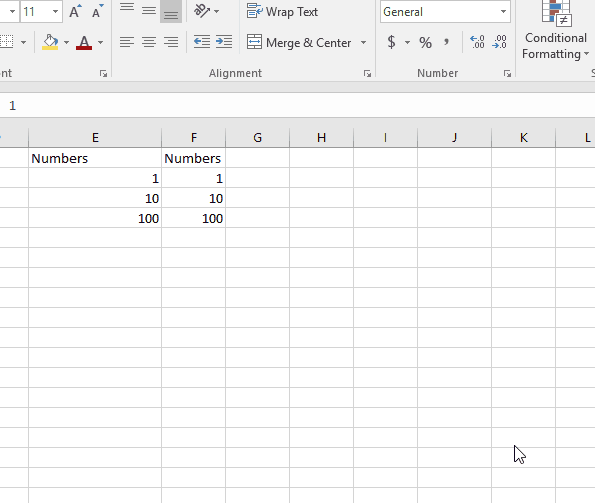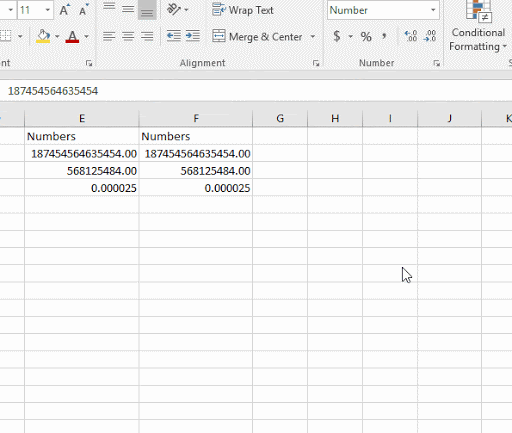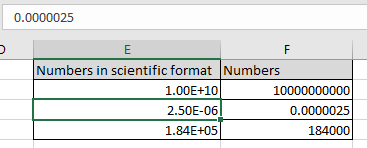# Perform Scientific formatting in Excel

The Scientific format displays a number in exponential notation, replacing part of the number with E+n, in which E (exponent) multiplies the preceding number by 10 to the nth power.

In simple words, sometimes working with mathematical operations on a sheet. We need numbers in Scientific format. Excel allows us to convert the format of the cell to scientific format.

For example, a 2-decimal scientific format displays 12345678901 as 1.23E+10, which is 1.23 times 10 to the 10th power.

Let’s understand this function using it an example.

Here we have 3 multiples of 10. Let’s see how to convert them into the scientific format.As you can see, we can convert numbers from general to scientific formatYou can convert multiples to 10 and decimal values to scientific format. When you convert a decimal value to scientific format, the number gets displayed in E-n format.

Now we will understand how to write in scientific format

Write 10^10 in scientific

1.00E+10

It will be displayed as 1.00E+10 in the cell.
Write 0.0000025 in scientific

2.50E-06

It will be displayed as 2.50E-06 in the cell.

Write 184000 in scientific

1.84E+05

It will be displayed as 1.84E+05 in the cell.

The above 3 representation of scientific format of numbers, shown in the snapshot below.Here cells have been converted to scientific format & written in Excel

Hope you understood how to convert into scientific format & written in Excel. Explore more articles on Excel cell formatting here. Please feel free to state your query or feedback for the above article.

Related Articles:

How to Use the Custom Format Cell in Excel

Excel convert decimal seconds into time format

Convert Text To Number Excel

Adding Zeros in front of the number in Excel

Convert month name to serial number in Excel

Popular Articles

50 Excel Shortcut to Increase Your Productivity : Get faster at your task. These 50 shortcuts will make you work even faster on Excel.

How to use the VLOOKUP Function in Excel : This is one of the most used and popular functions of excel that is used to lookup value from different ranges and sheets.

How to use the COUNTIF function in Excel : Count values with conditions using this amazing function. You don't need to filter your data to count specific values. Countif function is essential to prepare your dashboard.

How to use the SUMIF Function in Excel : This is another dashboard essential function. This helps you sum up values on specific conditions.

Terms and Conditions of use

The applications/code on this site are distributed as is and without warranties or liability. In no event shall the owner of the copyrights, or the authors of the applications/code be liable for any loss of profit, any problems or any damage resulting from the use or evaluation of the applications/code.